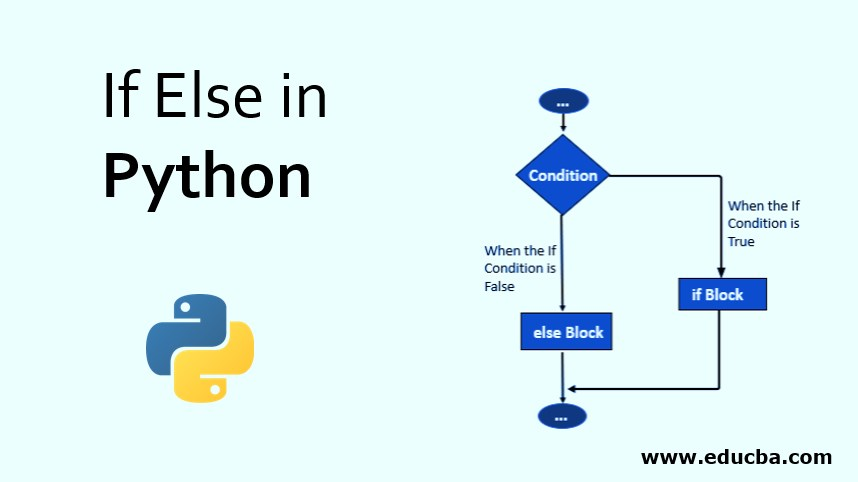28/05/2023Python Condition and if statement

Python programming language has used conditions in while and if statements that contain many operators, not compressions.

In this article, we will learn to create decision-making statements with python programming language using different forms of all kinds of statements.

Python always supported logical conditions from mathematics. These statements used several looks like if statements and loops. Always remember if statements are used by (IF) keywords.

That likes-

Equals – x == y

Not – equal- x != y

Less than – x < y

Less than or equal to – x <= y

Greater than – x > y

Greater than or equal to – x >= y

Example of If statements –

Suppose x= 55 and y = 155, here if statement include that like

X = 55

Y = 155

If y> x :

print(“y is greater than x”)

The output of these if statements-

Above the example of these, here used two variables x and y, and also used if statements to test y is greater than x. Here, x = 55 and y = 155 so that we know 155 is greater than 55. Which we print the if statements. In this example we are able to learn if statements are used in making decisions on mathematics.

## What if- else statements of the python programming language?

When you want to make a decision on that points you need to use if-else statements to define an exact value. When we want to excite code only if a certain condition is satisfied.

If -else condition is used mainly making a decision on the python programming language.

## Here we learn all Python Condition and if statementthat help to make decisions.

That provides below-

1. If statements
2. If- Else statements
3. Elif statements
4. Nested if statements

In this article, I explain all of the above statements and real-time examples of these conditions. So follow these attentively-

### # If statements

If statements one of the most commonly used conditional statements of the python programming language. It defines which include statement-making decisions or not. First of all it checks a condition, if the condition  is true then the set of instant inside of code if block will be executed otherwise not.

If condition evaluate boolean expression block on code when boolean expression is true

Syntax of these if condition example-

If ( EXPRESSION== TRUE ):

Block of code

Else:

Block of code

In this, statements of condition will be evaluated by boolean expression, if the condition is true, then the statements present inside of ‘if’ block will be executed, or if the condition is false then the statements present inside of ‘else’ block will be executed.

Flow chart of these statements of If

### # If – Else statements

If statements run code when the condition is TRUE otherwise not. When if the condition is not TRUE but if statements code run that called else statements.

Example of the syntax of if-else statements –

If (EXPIRATION == TRUE) :

Statement ( Body of the block )

else:

Statement ( Body of the block )

Flow chart of the statements of if-else

### #elif statements

Elif condition only used when need to check multiple conditions at a time that if given condition False. It is most similar to If – else condition, some different of the ‘elif’ statements check the condition but ‘else’ not checking any conditional.

Example of the syntax of elif conditions

x=1

If x >10 :

print(“x is greater than 10!”)

Elif x <10 :

print(“x is less than 10!”)

Elif x < 20 :

print(“x greater than 20!‘)

Else :

print(“x is equal to 10!”)

The output of these statements-

In the example, if statements of the first test specific conditions and elif two-block works alternatively and last else statements find out its solution point that showing up all previous statements have not met these conditions.

### #Nested If statements

When we see if…elif…else statements inside other if … elif … else statements that we called nested if statements of python. Any decimal or number can be this structure at this point.

Our previous article we learned indentation that can be figured out of nesting levels.

Example of python nested if statements-

num=float(input( “inter a number :” ))

if num >= 0:

if num == 0:

print(“Zero”)

else:

print (“Positive number”)

else:

print(“Negative number”)

The output of the nested 3 condition –

Elif statements contain a structured form of a ladder that basically works to test multiple expressions. Elif ladder statements view including all statements at a time then it creates an expression that works total point of view.

Example of the elif ladder statements-

my_marks = 95

if (my_marks < 30):

print(“Sorry to say that!, You failed the exam”)

elif(my_marks > 60 and my_marks > 100):

print(“Passed in First class”)

else:

print(“Passed in First class with distinction”)

Output of the statements-

In this article on python conditions and statements, I try to teach you that control flow in the execution program. Here I explain if, if-else, elif, if-elif-else, nested, elif ladder statements. Read carefully to know more about conditional statements. Hopefully, it’s very helpful if you want to become a python expert.

error: Content is protected !!